# TensorFlow RNN Cell源码解析

+关注继续查看

## RNN

RNN，循环神经网络，Recurrent Neural Networks。人们思考问题往往不是从零开始的，比如阅读时我们对每个词的理解都会依赖于前面看到的一些信息，而不是把前面看的内容全部抛弃再去理解某处的信息。应用到深度学习上面，如果我们想要学习去理解一些依赖上文的信息，RNN 便可以做到，它有一个循环的操作，可以使其可以保留之前学习到的内容。

RNN 的结构如下：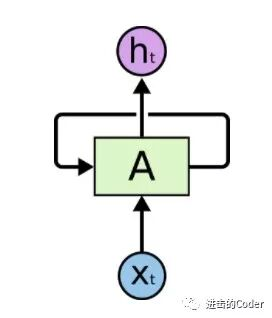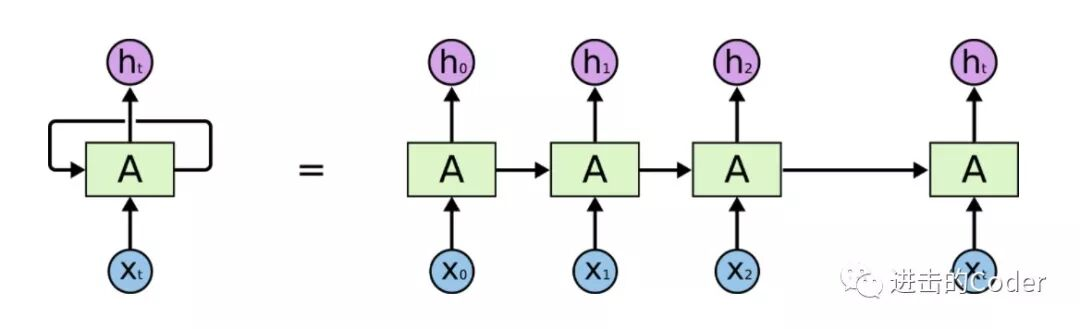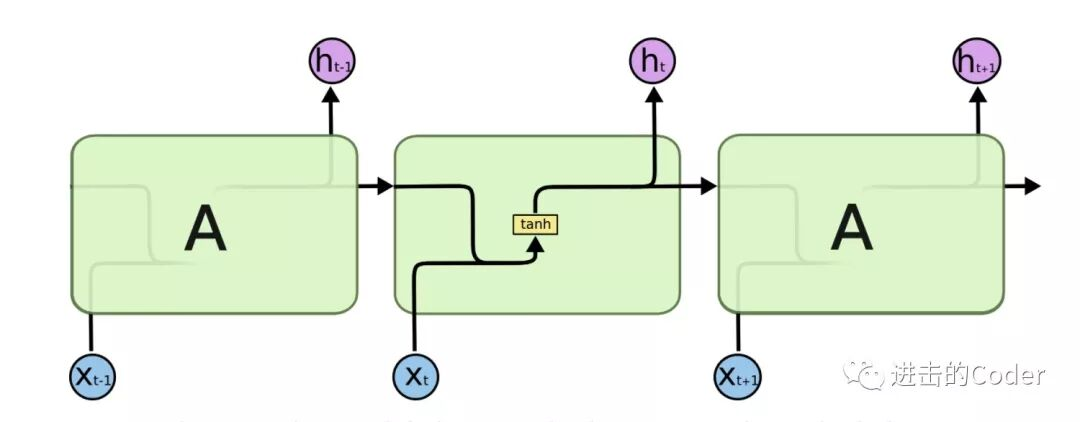TensorFlow 实现 RNN Cell 的位置在 python/ops/rnn_cell_impl.py，首先其实现了一个 RNNCell 类，继承了 Layer 类，其内部有三个比较重要的方法，state_size()、output_size()、__call__() 方法，其中 state_size() 和 output_size() 方法设置为类属性，可以当做属性来调用，实现如下：

@property

def state_size(self):

"""size(s) of state(s) used by this cell.

It can be represented by an Integer, a TensorShape or a tuple of Integers

or TensorShapes.

"""

raise NotImplementedError("Abstract method")

@property

def output_size(self):

"""Integer or TensorShape: size of outputs produced by this cell."""

raise NotImplementedError("Abstract method")

def __call__(self, inputs, state, scope=None):

if scope is not None:

with vs.variable_scope(scope,

custom_getter=self._rnn_get_variable) as scope:

return super(RNNCell, self).__call__(inputs, state, scope=scope)

else:

with vs.variable_scope(vs.get_variable_scope(),

custom_getter=self._rnn_get_variable):

return super(RNNCell, self).__call__(inputs, state)

def call(self, inputs, **kwargs):

return inputs

class BasicRNNCell(RNNCell):

"""The most basic RNN cell.

Args:

num_units: int, The number of units in the RNN cell.

activation: Nonlinearity to use.  Default: tanh.

reuse: (optional) Python boolean describing whether to reuse variables

in an existing scope.  If not True, and the existing scope already has

the given variables, an error is raised.

"""

def __init__(self, num_units, activation=None, reuse=None):

super(BasicRNNCell, self).__init__(_reuse=reuse)

self._num_units = num_units

self._activation = activation or math_ops.tanh

self._linear = None

@property

def state_size(self):

return self._num_units

@property

def output_size(self):

return self._num_units

def call(self, inputs, state):

"""Most basic RNN: output = new_state = act(W * input + U * state + B)."""

if self._linear is None:

self._linear = _Linear([inputs, state], self._num_units, True)

output = self._activation(self._linear([inputs, state]))

return output, output

def __call__(self, args):

if not self._is_sequence:

args = [args]

if len(args) == 1:

res = math_ops.matmul(args, self._weights)

else:

res = math_ops.matmul(array_ops.concat(args, 1), self._weights)

if self._build_bias:

return res

import tensorflow as tf

cell = tf.nn.rnn_cell.BasicRNNCell(num_units=128)

print(cell.state_size)

inputs = tf.placeholder(tf.float32, shape=[32, 100])

h0 = cell.zero_state(32, tf.float32)

output, h1 = cell(inputs=inputs, state=h0)

print(output, output.shape)

print(h1, h1.shape)

128

Tensor("basic_rnn_cell/Tanh:0", shape=(32, 128), dtype=float32) (32, 128)

Tensor("basic_rnn_cell/Tanh:0", shape=(32, 128), dtype=float32) (32, 128)

## LSTM

RNNs 的出现，主要是因为它们能够把以前的信息联系到现在，从而解决现在的问题。比如，利用前面的信息，能够帮助我们理解当前的内容。LSTM，Long Short Term Memory Networks，是 RNN 的一个变种，经试验它可以用来解决更多问题，并取得了非常好的效果。

LSTM Cell 的结构如下：LSTMs 最关键的地方在于 Cell 的状态 和 结构图上面的那条横穿的水平线。

Cell 状态的传输就像一条传送带，向量从整个 Cell 中穿过，只是做了少量的线性操作。这种结构能够很轻松地实现信息从整个 Cell 中穿过而不做改变。### 遗忘门（Forget Gate）### 传入门（Input Gate）### 输出门 （Output Gate）def __init__(self, num_units, forget_bias=1.0,

state_is_tuple=True, activation=None, reuse=None):

super(BasicLSTMCell, self).__init__(_reuse=reuse)

if not state_is_tuple:

logging.warn("%s: Using a concatenated state is slower and will soon be "

"deprecated.  Use state_is_tuple=True.", self)

self._num_units = num_units

self._forget_bias = forget_bias

self._state_is_tuple = state_is_tuple

self._activation = activation or math_ops.tanh

self._linear = None

@property

def state_size(self):

return (LSTMStateTuple(self._num_units, self._num_units)

if self._state_is_tuple else 2 * self._num_units)

@property

def output_size(self):

return self._num_units

def call(self, inputs, state):

"""Long short-term memory cell (LSTM).

Args:

inputs: 2-D tensor with shape [batch_size x input_size].

state: An LSTMStateTuple of state tensors, each shaped

[batch_size x self.state_size], if state_is_tuple has been set to

True.  Otherwise, a Tensor shaped

[batch_size x 2 * self.state_size].

Returns:

A pair containing the new hidden state, and the new state (either a

LSTMStateTuple or a concatenated state, depending on

state_is_tuple).

"""

sigmoid = math_ops.sigmoid

# Parameters of gates are concatenated into one multiply for efficiency.

if self._state_is_tuple:

c, h = state

else:

c, h = array_ops.split(value=state, num_or_size_splits=2, axis=1)

if self._linear is None:

self._linear = _Linear([inputs, h], 4 * self._num_units, True)

# i = input_gate, j = new_input, f = forget_gate, o = output_gate

i, j, f, o = array_ops.split(

value=self._linear([inputs, h]), num_or_size_splits=4, axis=1)

new_c = (

c * sigmoid(f + self._forget_bias) + sigmoid(i) * self._activation(j))

new_h = self._activation(new_c) * sigmoid(o)

if self._state_is_tuple:

new_state = LSTMStateTuple(new_c, new_h)

else:

new_state = array_ops.concat([new_c, new_h], 1)

return new_h, new_state

if self._state_is_tuple:

c, h = state

else:

c, h = array_ops.split(value=state, num_or_size_splits=2, axis=1)

i, j, f, o = array_ops.split(value=self._linear([inputs, h]), num_or_size_splits=4, axis=1)

new_c = (c * sigmoid(f + self._forget_bias) + sigmoid(i) * self._activation(j))

new_h = self._activation(new_c) * sigmoid(o)

if self._state_is_tuple:

new_state = LSTMStateTuple(new_c, new_h)

else:

new_state = array_ops.concat([new_c, new_h], 1)

return new_h, new_state

import tensorflow as tf

cell = tf.nn.rnn_cell.BasicLSTMCell(num_units=128)

print(cell.state_size)

inputs = tf.placeholder(tf.float32, shape=(32, 100))

h0 = cell.zero_state(32, tf.float32)

output, h1 = cell(inputs=inputs, state=h0)

print(h1)

print(h1.h, h1.h.shape)

print(h1.c, h1.c.shape)

print(output, output.shape)

LSTMStateTuple(c=128, h=128)

LSTMStateTuple(c=<tf.Tensor 'add_1:0' shape=(32, 128) dtype=float32>, h=<tf.Tensor 'mul_2:0' shape=(32, 128) dtype=float32>)

Tensor("mul_2:0", shape=(32, 128), dtype=float32) (32, 128)

Tensor("add_1:0", shape=(32, 128), dtype=float32) (32, 128)

Tensor("mul_2:0", shape=(32, 128), dtype=float32) (32, 128)### GRU

GRU，Gated Recurrent Unit，在 GRU 中，只有两个门：重置门（Reset Gate）和更新门（Update Gate）。同时在这个结构中，把 Ct 和隐藏状态进行了合并，整体结构比标准的 LSTM 结构要简单，而且这个结构后来也非常流行。class GRUCell(RNNCell):

"""Gated Recurrent Unit cell (cf. http://arxiv.org/abs/1406.1078).

Args:

num_units: int, The number of units in the GRU cell.

activation: Nonlinearity to use.  Default: tanh.

reuse: (optional) Python boolean describing whether to reuse variables

in an existing scope.  If not True, and the existing scope already has

the given variables, an error is raised.

kernel_initializer: (optional) The initializer to use for the weight and

projection matrices.

bias_initializer: (optional) The initializer to use for the bias.

"""

def __init__(self,

num_units,

activation=None,

reuse=None,

kernel_initializer=None,

bias_initializer=None):

super(GRUCell, self).__init__(_reuse=reuse)

self._num_units = num_units

self._activation = activation or math_ops.tanh

self._kernel_initializer = kernel_initializer

self._bias_initializer = bias_initializer

self._gate_linear = None

self._candidate_linear = None

@property

def state_size(self):

return self._num_units

@property

def output_size(self):

return self._num_units

def call(self, inputs, state):

"""Gated recurrent unit (GRU) with nunits cells."""

if self._gate_linear is None:

bias_ones = self._bias_initializer

if self._bias_initializer is None:

bias_ones = init_ops.constant_initializer(1.0, dtype=inputs.dtype)

with vs.variable_scope("gates"):  # Reset gate and update gate.

self._gate_linear = _Linear(

[inputs, state],

2 * self._num_units,

True,

bias_initializer=bias_ones,

kernel_initializer=self._kernel_initializer)

value = math_ops.sigmoid(self._gate_linear([inputs, state]))

r, u = array_ops.split(value=value, num_or_size_splits=2, axis=1)

r_state = r * state

if self._candidate_linear is None:

with vs.variable_scope("candidate"):

self._candidate_linear = _Linear(

[inputs, r_state],

self._num_units,

True,

bias_initializer=self._bias_initializer,

kernel_initializer=self._kernel_initializer)

c = self._activation(self._candidate_linear([inputs, r_state]))

new_h = u * state + (1 - u) * c

return new_h, new_h

value = math_ops.sigmoid(self._gate_linear([inputs, state]))

r, u = array_ops.split(value=value, num_or_size_splits=2, axis=1)

r_state = r * state

c = self._activation(self._candidate_linear([inputs, r_state]))

new_h = u * state + (1 - u) * c

return new_h, new_h

import tensorflow as tf

cell = tf.nn.rnn_cell.GRUCell(num_units=128)

print(cell.state_size)

inputs = tf.placeholder(tf.float32, shape=[32, 100])

h0 = cell.zero_state(32, tf.float32)

output, h1 = cell(inputs=inputs, state=h0)

print(output, output.shape)

print(h1, h1.shape)

128

Tensor("gru_cell/add:0", shape=(32, 128), dtype=float32) (32, 128)

Tensor("gru_cell/add:0", shape=(32, 128), dtype=float32) (32, 128)

## 结语76 0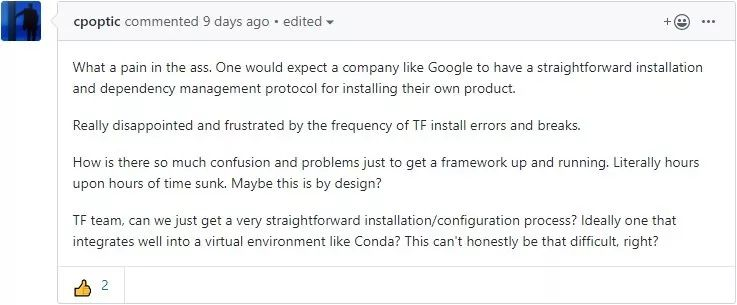326 0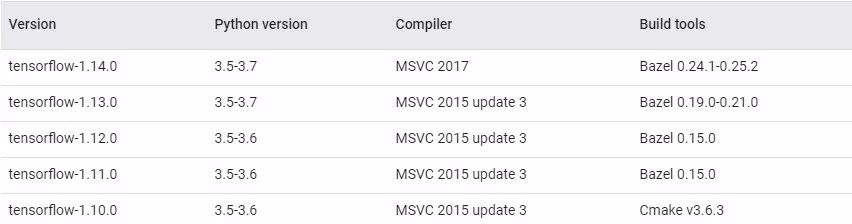103 0TensorFlow系列专题（十一）：RNN的应用及注意力模型
4013 0TensorFlow系列专题（九）：常用RNN网络结构及依赖优化问题
2079 0TensorFlow系列专题（八）：七步带你实现RNN循环神经网络小示例
1650 0TensorFlow系列专题（七）：一文综述RNN循环神经网络
2309 05777 0YJango：TensorFlow高层API Custom Estimator建立CNN+RNN的演示
TensorFlow 目前是最为常用的深度学习框架。然而从头编写整个机器学习流程却是一件繁琐的工作。虽然上有 Keras 帮助减轻代码量，但这次 TensorFlow 自己提供了高阶 API estimators。
1678 0885 0
Chin201812810